Markov Chain Monte Carlo Method

The Markov chain Monte Carlo (MCMC) method is a general simulation method for sampling from posterior distributions and computing posterior quantities of interest. MCMC methods sample successively from a target distribution. Each sample depends on the previous one, hence the notion of the Markov chain. A Markov chain is a sequence of random variables,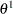,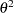,, for which the random variable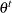depends on all previous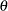s only through its immediate predecessor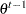. You can think of a Markov chain applied to sampling as a mechanism that traverses randomly through a target distribution without having any memory of where it has been. Where it moves next is entirely dependent on where it is now.

Monte Carlo, as in Monte Carlo integration, is mainly used to approximate an expectation by using the Markov chain samples. In the simplest version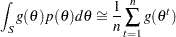where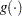is a function of interest andare samples from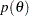on its support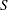. This approximates the expected value of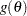. The earliest reference to MCMC simulation occurs in the physics literature. Metropolis and Ulam (1949) and Metropolis et al. (1953) describe what is known as the Metropolis algorithm (see the section Metropolis and Metropolis-Hastings Algorithms). The algorithm can be used to generate sequences of samples from the joint distribution of multiple variables, and it is the foundation of MCMC. Hastings (1970) generalized their work, resulting in the Metropolis-Hastings algorithm. Geman and Geman (1984) analyzed image data by using what is now called Gibbs sampling (see the section Gibbs Sampler). These MCMC methods first appeared in the mainstream statistical literature in Tanner and Wong (1987).

The Markov chain method has been quite successful in modern Bayesian computing. Only in the simplest Bayesian models can you recognize the analytical forms of the posterior distributions and summarize inferences directly. In moderately complex models, posterior densities are too difficult to work with directly. With the MCMC method, it is possible to generate samples from an arbitrary posterior density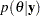and to use these samples to approximate expectations of quantities of interest. Several other aspects of the Markov chain method also contributed to its success. Most importantly, if the simulation algorithm is implemented correctly, the Markov chain is guaranteed to converge to the target distributionunder rather broad conditions, regardless of where the chain was initialized. In other words, a Markov chain is able to improve its approximation to the true distribution at each step in the simulation. Furthermore, if the chain is run for a very long time (often required), you can recoverto any precision. Also, the simulation algorithm is easily extensible to models with a large number of parameters or high complexity, although the "curse of dimensionality" often causes problems in practice.

Properties of Markov chains are discussed in Feller (1968), Breiman (1968), and Meyn and Tweedie (1993). Ross (1997) and Karlin and Taylor (1975) give a non-measure-theoretic treatment of stochastic processes, including Markov chains. For conditions that govern Markov chain convergence and rates of convergence, see Amit (1991), Applegate, Kannan, and Polson (1990), Chan (1993), Geman and Geman (1984), Liu, Wong, and Kong (1991a, 1991b), Rosenthal (1991a, 1991b), Tierney (1994), and Schervish and Carlin (1992). Besag (1974) describes conditions under which a set of conditional distributions gives a unique joint distribution. Tanner (1993), Gilks, Richardson, and Spiegelhalter (1996), Chen, Shao, and Ibrahim (2000), Liu (2001), Gelman et al. (2004), Robert and Casella (2004), and Congdon (2001, 2003, 2005) provide both theoretical and applied treatments of MCMC methods. You can also see the section A Bayesian Reading List for a list of books with varying levels of difficulty of treatment of the subject and its application to Bayesian statistics.

### Metropolis and Metropolis-Hastings Algorithms

The Metropolis algorithm is named after its inventor, the American physicist and computer scientist Nicholas C. Metropolis. The algorithm is simple but practical, and it can be used to obtain random samples from any arbitrarily complicated target distribution of any dimension that is known up to a normalizing constant.

Suppose you want to obtain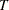samples from a univariate distribution with probability density function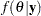. Supposeis theth sample from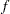. To use the Metropolis algorithm, you need to have an initial value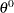and a symmetric proposal density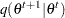. For the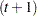th iteration, the algorithm generates a sample from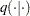based on the current sample, and it makes a decision to either accept or reject the new sample. If the new sample is accepted, the algorithm repeats itself by starting at the new sample. If the new sample is rejected, the algorithm starts at the current point and repeats. The algorithm is self-repeating, so it can be carried out as long as required. In practice, you have to decide the total number of samples needed in advance and stop the sampler after that many iterations have been completed.

Suppose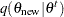is a symmetric distribution. The proposal distribution should be an easy distribution from which to sample, and it must be such that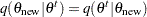, meaning that the likelihood of jumping to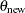fromis the same as the likelihood of jumping back tofrom. The most common choice of the proposal distribution is the normal distribution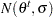with a fixed
. The Metropolis algorithm can be summarized as follows:

1. Set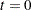. Choose a starting point. This can be an arbitrary point as long as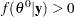.

2. Generate a new sample,, by using the proposal distribution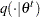.

3. Calculate the following quantity: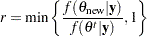4. Samplefrom the uniform distribution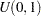.

5. Set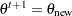if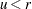; otherwise set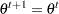.

6. Set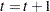. If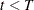, the number of desired samples, return to step 2. Otherwise, stop.

Note that the number of iteration keeps increasing regardless of whether a proposed sample is accepted.

This algorithm defines a chain of random variates whose distribution will converge to the desired distribution, and so from some point forward, the chain of samples is a sample from the distribution of interest. In Markov chain terminology, this distribution is called the stationary distribution of the chain, and in Bayesian statistics, it is the posterior distribution of the model parameters. The reason that the Metropolis algorithm works is beyond the scope of this documentation, but you can find more detailed descriptions and proofs in many standard textbooks, including Roberts (1996) and Liu (2001). The random-walk Metropolis algorithm is used in the MCMC procedure.

You are not limited to a symmetric random-walk proposal distribution in establishing a valid sampling algorithm. A more general form, the Metropolis-Hastings (MH) algorithm, was proposed by Hastings (1970). The MH algorithm uses an asymmetric proposal distribution: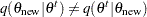. The difference in its implementation comes in calculating the ratio of densities: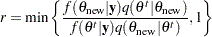Other steps remain the same.

The extension of the Metropolis algorithm to a higher-dimensional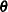is straightforward. Suppose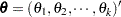is the parameter vector. To start the Metropolis algorithm, select an initial value for each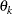and use a multivariate version of proposal distribution, such as a multivariate normal distribution, to select a-dimensional new parameter. Other steps remain the same as those previously described, and this Markov chain eventually converges to the target distribution of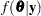. Chib and Greenberg (1995) provide a useful tutorial on the algorithm.

### Gibbs Sampler

The Gibbs sampler, named by Geman and Geman (1984) after the American physicist Josiah W. Gibbs, is a special case of the Metropolis and Metropolis-Hastings Algorithms in which the proposal distributions exactly match the posterior conditional distributions and proposals are accepted 100% of the time. Gibbs sampling requires you to decompose the joint posterior distribution into full conditional distributions for each parameter in the model and then sample from them. The sampler can be efficient when the parameters are not highly dependent on each other and the full conditional distributions are easy to sample from. Some researchers favor this algorithm because it does not require an instrumental proposal distribution as Metropolis methods do. However, while deriving the conditional distributions can be relatively easy, it is not always possible to find an efficient way to sample from these conditional distributions.

Suppose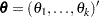is the parameter vector,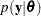is the likelihood, and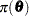is the prior distribution. The full posterior conditional distribution of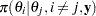is proportional to the joint posterior density; that is,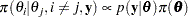For instance, the one-dimensional conditional distribution of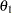given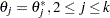, is computed as the following: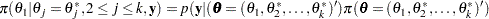The Gibbs sampler works as follows:

1. Set, and choose an arbitrary initial value of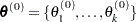.

2. Generate each component ofas follows:

• draw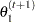from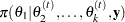• draw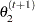from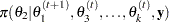• ...

• draw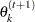from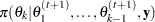3. Set. If, the number of desired samples, return to step 2. Otherwise, stop.

The name "Gibbs" was introduced by Geman and Geman (1984). Gelfand et al. (1990) first used Gibbs sampling to solve problems in Bayesian inference. See Casella and George (1992) for a tutorial on the sampler. The GENMOD, LIFEREG, and PHREG procedures update parameters using the Gibbs sampler.

### Adaptive Rejection Sampling Algorithm

The GENMOD, LIFEREG, and PHREG procedures use the adaptive rejection sampling (ARS) algorithm to sample parameters sequentially from their univariate full conditional distributions. The ARS algorithm is a rejection algorithm that was originally proposed by Gilks and Wild (1992). Given a log-concave density (the log of the density is concave), you can construct an envelope to the density by using linear segments. You then use the linear segment envelope as a proposal density (it becomes a piecewise exponential density on the original scale and is easy to generate samplers from) in the rejection sampling. The log-concavity condition is met in some of the models fit by the procedures. For example, the posterior densities for the regression parameters in the generalized linear models are log-concave under flat priors. When this condition fails, the ARS algorithm calls for an additional Metropolis-Hastings step (Gilks, Best, and Tan; 1995), and the modified algorithm becomes the adaptive rejection metropolis sampling (ARMS) algorithm. The GENMOD, LIFEREG, and PHREG procedures can recognize whether a model is log-concave and select the appropriate sampler for the problem at hand.

The GENMOD, LIFEREG, and PHREG procedures implement the ARMS algorithm based on code kindly provided by Walter R. Gilks, University of Leeds (Gilks; 2003), to obtain posterior samples. For a detailed description and explanation of the algorithm, see Gilks and Wild (1992) and Gilks, Best, and Tan (1995).

### Independence Sampler

Another type of Metropolis algorithm is the "independence" sampler. It is called the independence sampler because the proposal distribution in the algorithm does not depend on the current point as it does with the random-walk Metropolis algorithm. For this sampler to work well, you want to have a proposal distribution that mimics the target distribution and have the acceptance rate be as high as possible.

1. Set. Choose a starting point. This can be an arbitrary point as long as.

2. Generate a new sample,, by using the proposal distribution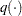. The proposal distribution does not depend on the current value of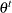.

3. Calculate the following quantity: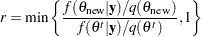4. Samplefrom the uniform distribution.

5. Setif; otherwise set.

6. Set. If, the number of desired samples, return to step 2. Otherwise, stop.

A good proposal density should have thicker tails than those of the target distribution. This requirement sometimes can be difficult to satisfy especially in cases where you do not know what the target posterior distributions are like. In addition, this sampler does not produce independent samples as the name seems to imply, and sample chains from independence samplers can get stuck in the tails of the posterior distribution if the proposal distribution is not chosen carefully. The MCMC procedure uses the independence sampler.

### Gamerman Algorithm

The Gamerman algorithm, named after the inventor Dani Gamerman is a special case of the Metropolis and Metropolis-Hastings Algorithms in which the proposal distribution is derived from one iteration of the iterative weighted least squares (IWLS) algorithm. As the name suggests, a weighted least squares algorithm is carried out inside an iteration loop. For each iteration, a set of weights for the observations is used in the least squares fit. The weights are constructed by applying a weight function to the current residuals. The proposal distribution uses the current iteration’s values of the parameters to form the proposal distribution from which to generate a proposed random value (Gamerman; 1997).

The multivariate sampling algorithm is simple but practical, and can be used to obtain random samples from the posterior distribution of the regression parameters in a generalized linear model (GLM). See Generalized Linear Regression for further details on generalized linear regression models. See McCullagh and Nelder (1989) for a discussion of transformed observations and diagonal matrix of weights pertaining to IWLS.

The GENMOD procedure uses the Gamerman algorithm to sample parameters from their multivariate posterior conditional distributions. For a detailed description and explanation of the algorithm, see Gamerman (1997).

### Burn-in, Thinning, and Markov Chain Samples

Burn-in refers to the practice of discarding an initial portion of a Markov chain sample so that the effect of initial values on the posterior inference is minimized. For example, suppose the target distribution is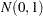and the Markov chain was started at the value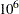. The chain might quickly travel to regions around 0 in a few iterations. However, including samples around the valuein the posterior mean calculation can produce substantial bias in the mean estimate. In theory, if the Markov chain is run for an infinite amount of time, the effect of the initial values decreases to zero. In practice, you do not have the luxury of infinite samples. In practice, you assume that afteriterations, the chain has reached its target distribution and you can throw away the early portion and use the good samples for posterior inference. The value ofis the burn-in number.

With some models you might experience poor mixing (or slow convergence) of the Markov chain. This can happen, for example, when parameters are highly correlated with each other. Poor mixing means that the Markov chain slowly traverses the parameter space (see the section Visual Analysis via Trace Plots for examples of poorly mixed chains) and the chain has high dependence. High sample autocorrelation can result in biased Monte Carlo standard errors. A common strategy is to thin the Markov chain in order to reduce sample autocorrelations. You thin a chain by keeping everyth simulated draw from each sequence. You can safely use a thinned Markov chain for posterior inference as long as the chain converges. It is important to note that thinning a Markov chain can be wasteful because you are throwing away a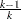fraction of all the posterior samples generated. MacEachern and Berliner (1994) show that you always get more precise posterior estimates if the entire Markov chain is used. However, other factors, such as computer storage or plotting time, might prevent you from keeping all samples.

To use the GENMOD, LIFEREG, MCMC, and PHREG procedures, you need to determine the total number of samples to keep ahead of time. This number is not obvious and often depends on the type of inference you want to make. Mean estimates do not require nearly as many samples as small-tail percentile estimates. In most applications, you might find that keeping a few thousand iterations is sufficient for reasonably accurate posterior inference. In all four procedures, the relationship between the number of iterations requested, the number of iterations kept, and the amount of thinning is as follows: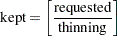where
is the rounding operator.